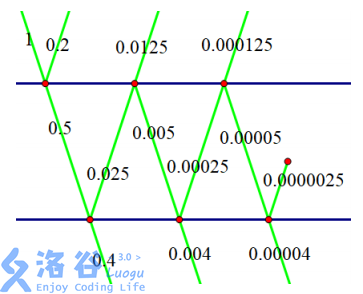P5323 [BJOI2019]光线

• 235通过
• 314提交
• 题目提供者 NaCly_Fish
• 评测方式 云端评测
• 标签 数论,数学 各省省选 2019 北京 O2优化
• 难度 省选/NOI-
• 时空限制 1000ms / 512MB
• 提示：收藏到任务计划后，可在首页查看。

题目描述

当一束光打到一层玻璃上时，有一定比例的光会穿过这层玻璃，一定比例的光会被反射回去，剩下的光被玻璃吸收。

设对于任意$x$，有$x* a_i\%$单位的光会穿过它，有$x* b_i\%$的会被反射回去。
现在$n$层玻璃叠在一起，有$1$单位的光打到第$1$层玻璃上，那么有多少单位的光能穿过所有$n$层玻璃呢？

输入输出格式

输入格式：

第一行一个正整数$n$，表示玻璃层数。
接下来$n$行，每行两个非负整数$a_i,b_i$，表示第$i$层玻璃的透光率和反射率。

输出格式：

输出一行一个整数，表示穿透所有玻璃的光对$10^9 + 7$取模的结果。
可以证明，答案一定为有理数。设答案为$a/b$($a$和$b$是互质的正整数)，你输出的答案为$x$，你需要保证$a\equiv bx \space (\text{mod }10^9 + 7)$。

输入输出样例

输入样例#1： 复制
2
50 20
80 5
输出样例#1： 复制
858585865
输入样例#2： 复制
3
1 2
3 4
5 6
输出样例#2： 复制
843334849

说明

样例1解释：如图，光线从左上角打进来，有 $0.5$ 单位的光穿过第 $1$ 层玻璃，有 $0.2$ 单位的光被反射回去。这 $0.5$ 单位的光有 $0.4$ 单位穿过第 $2$ 层玻璃，有 $0.025$ 单位的光被反射回去。这 $0.025$ 单位的光有 $0.0125$ 单位穿过第 $1$ 层玻璃，有 $0.005$ 单位的光被反射回去。这 $0.005$ 单位的光有 $0.004$ 单位穿过第 $2$ 层玻璃……于是，穿过两层玻璃的光一共有$0.40404... = 40/99$单位。在模$10^9+7$意义下等于$858585865$。

数据范围：
对于$5\%$的数据，$n=1$
对于$20\%$的数据，$n\le 2$
对于$30\%$的数据，$n\le 3$
对于$50\%$的数据，$n\le 100$
对于$70\%$的数据，$n\le 3000$
对于$100\%$的数据：
$n\le 5\times 10^5$
$1\le a_i \le 100$
$0\le b_i \le 99$
$1\le a_i+b_i \le 100$
每组$a_i$和$b_i$在满足上述限制的整数中随机生成。

提示
标程仅供做题后或实在无思路时参考。
请自觉、自律地使用该功能并请对自己的学习负责。
如果发现恶意抄袭标程，将按照I类违反进行处理。